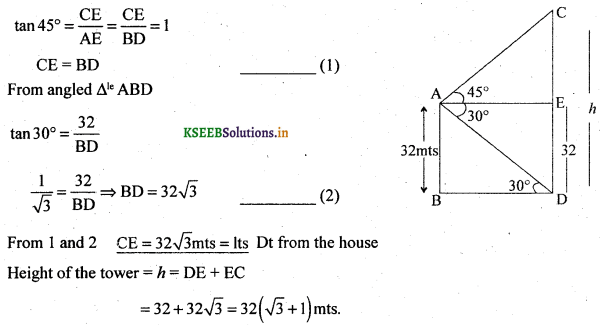# 2nd PUC Basic Maths Question Bank Chapter 13 Heights and Distances

Students can Download Basic Maths Question Bank Chapter 13 Heights and Distances Questions and Answers, Notes Pdf, 2nd PUC Basic Maths Question Bank with Answers helps you to revise the complete Karnataka State Board Syllabus and to clear all their doubts, score well in final exams.

## Karnataka 2nd PUC Basic Maths Question Bank Chapter 13 Heights and Distances

### 2nd PUC Basic Maths Heights and Distances Two or Three Marks Questions and Answers

Question 1.
The angle of elevation of the top of a tower at a distance 500 metres from its foot is 30°. Find the height of the tower.
Let O be the position of the observer. AB denote the height of the tower = h. From the rt angled triangle ABO. We get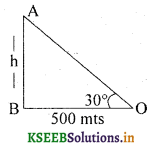Question 2.
What is the angle of elevation of the sun when the length of the shadow of a pole is
$$\frac{1}{\sqrt{3}}$$
Let O be the position of the observer.
AB denote the height of the Pole = h,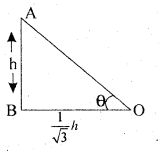From the rt angled Δle ABO, we getQuestion 3.
The angle of elevation of the top of a chimney at a distance of 100 meters from a foot is 30°. Find its height.
Let O be the position of the observer.
AB denote the height of the Chimney = h,
From the rt angled Δle ABO, we get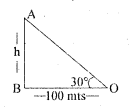$$\tan 30^{\circ}=\frac{h}{100} \Rightarrow \frac{1}{\sqrt{3}}=\frac{h}{100} \Rightarrow h=\frac{100 \sqrt{3}}{3}$$Question 4.
From a ship a most head 40 metres high the angle of depression: on of a boat is observed to be 45°. Find its distance from the ship.
Let AB be the mast head of a ship = 40 mts
O be the position of the boat
From the right angled Δle ABO$$\tan 45^{\circ}=\frac{40}{x} \Rightarrow 1=\frac{40}{x} \Rightarrow x=40$$
Its distance from the mast head = 40 mts.

Question 5.
Find the angle of elevation of the sun when the shadow of a tower 75 meters high is 25 $$\sqrt{3}$$ metres long.
Let AB be the height of the tower
From a right angled triangle ABO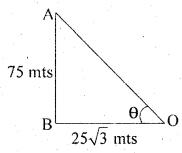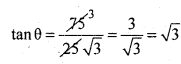⇒ θ = 60º

Question 6.
A kite flying at a height of h is tied to a thread which is 500m long. Assuming that there is no kink in the thrwd and it makes an angles of 30° with the ground. Find the height of the Kite.
AB = h be the height of the Kite
AO = 500 m
∴ From a right angled triangle ABO$$\sin 30^{\circ}=\frac{h}{500} \Rightarrow \frac{1}{2}=\frac{h}{500} \Rightarrow h=\frac{500}{2}=250 \mathrm{mts}$$
∴  The height of the kite = 250 mts.Question 7.
A ladder leaning against a wall makes an angle of 600 with the ground. The foot of the
ladder is 6m away from the wall. Find the length of the ladder.
From the right angled triangle
$$\cos 60^{\circ}=\frac{6}{\mathrm{AO}} \Rightarrow \frac{1}{2}=\frac{6}{\mathrm{AO}} \Rightarrow \mathrm{AO}=12 \mathrm{mts}$$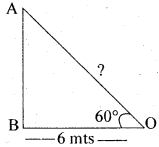The length of the ladder = 12 mts.

Question 8.
Find the angle of elevation of the sun’s rays from a point on the ground at a distance of
$$3 \sqrt{3} \mathrm{m}$$, from the foot of tower 3m high.
AB be the height of the pole
Let Ab = 3 mts, BO = $$3 \sqrt{3} \mathrm{m}$$ mts, θ = ?
From the right angled triangle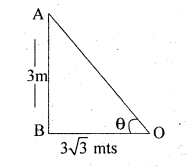We have⇒ θ = 30°
The angle of Elevation is 30°.

### 2nd PUC Basic Maths Heights and Distances Four or Five Marks Questions and Answers

Question 9.
The angles of elevation of the top of a tower from the base and the top of a building are 60° and 45°. The building is 20 meters high. Find the height of the tower.
AB = Building and CD is the tower
From a rt angled Δle ACD we haveQuestion 10.
The shadow of a tower standing on a level plane is found to be 50 metres longer when sun’s altitude is 30° than when it is 60°. Find the height of the tower.
AB = tower
From the Δle ABCQuestion 11.
An aeroplane when flying at a heights of 2000 metres passes; vertically above another plane at an instant when their angles of elevation from the same point of observation are 60° and 45° respectively. Find the distance between the aeroplans.Question 12.
From a point on the line joining the feet of two poles of equal heights, the angles of elevation of the tops of the poles are observed to be 30° and 60°. If the distance between the poles is a find (i) The height of the poles (ii) The position of the point of observation.
AB and CD are Two poles
O – pts of observation
From Δ ABO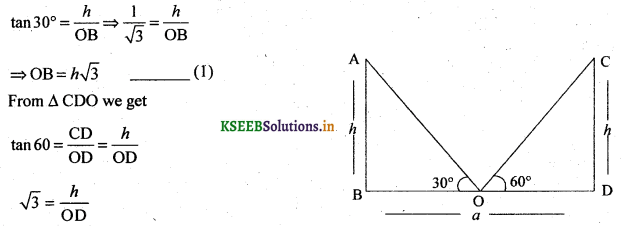Question 13.
The angles of elevation of the top of a tower from two points distant a and b (a < b) from its foot and the same straight line from it are 30° and 60°. Show that the height of the tower is  $$\sqrt{a b}$$
CD is the tower
From Rt angled Δle ACD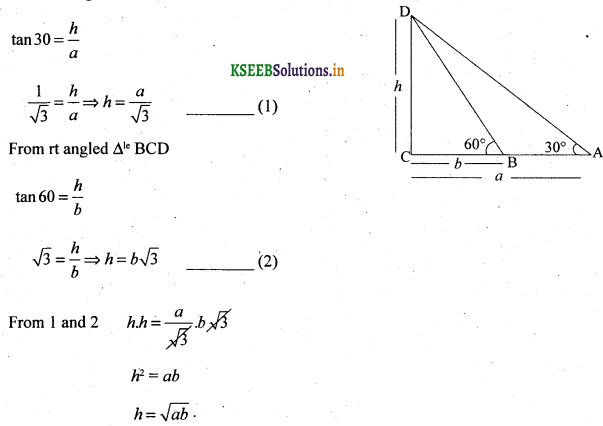Question 14.
A flag staff stands upon the top of a building. At a distance 20 meters the angles of elevation of the top of the flag staff and building are 60° and 30° respectively. Find the height of the flag staff.
AB = Building
From Δle ABC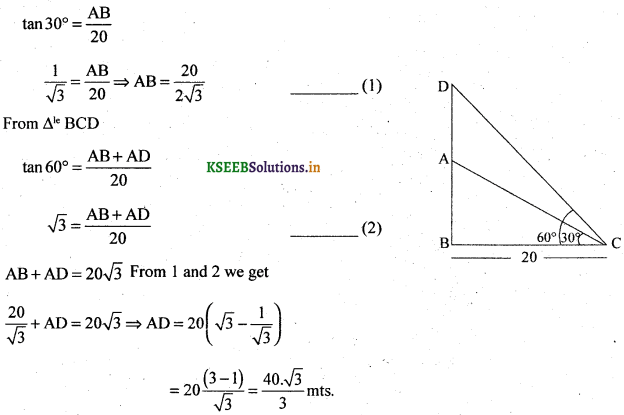Question 15.
From the top of a cliff. The angles of depression of two boats in the same vertical plane as the observer are 30° and 45°. If the distance between the boats is 100 metres. Find the height of the cliff.
AB is a cliff, C and D are
Positions of boats
From rt angled Δle ACE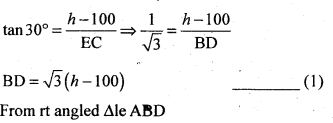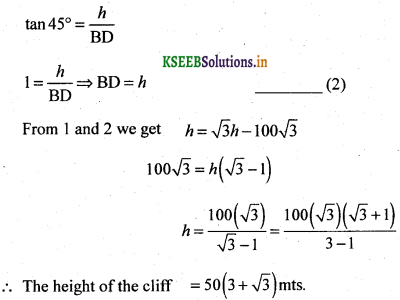Question 16.
From a point A due north of the tower, the elevation of the top of the tower is 60° from a point B due south, the elevation is 45°, if AB = 100 meters, show that the height of the tower is $$50 \sqrt{3}(\sqrt{3}-1)$$ metres.
A and B are position of observation
AB = 100 mts and CD is the hight of tower and CD = h
From rt angled Δle ACDQuestion 17.
A person at the top of a hill observes that the angles of depression of two consecutive kilometers stones on a road leading to the foot of the hill and in the same vertical plane containing the position of the observer are 30° and 60°. Find the height of the hill.
Let AB be the hill and AB = h
From rt angled Δle ABC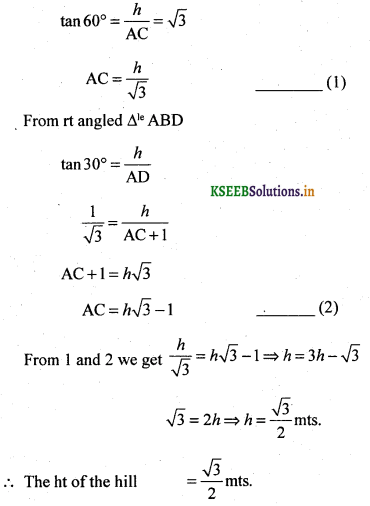Question 18.
Problem = the angles of elevation of the top of a tower fran the base and the top of a building are 60° & 45° the building is 32 meters high find the height of the tower.
AB is the tower
From rt angled Δle ABC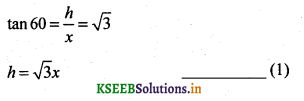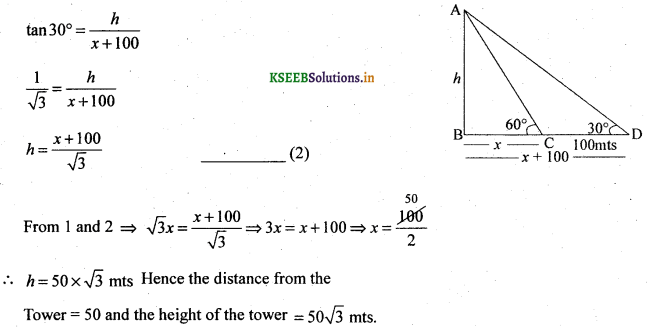Question 19.
The angle of elevation of a tower from a point on the ground is 30°. At a point on the horizontal line passing throught the foot of the tower and 100 meters nearer it, the angle of elevation is found to be 60°. Find the height of the tower and the distance of the first point from the tower.